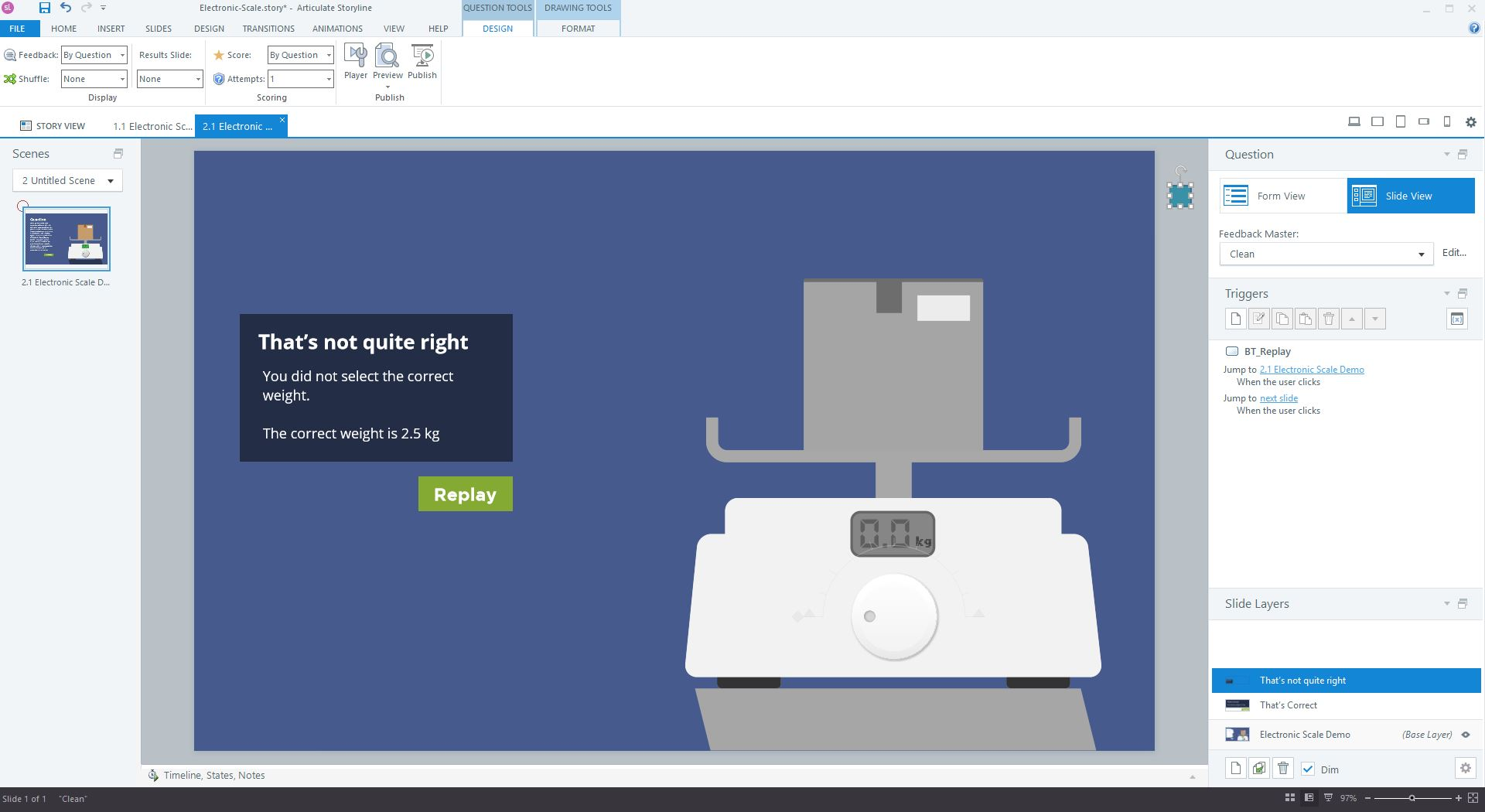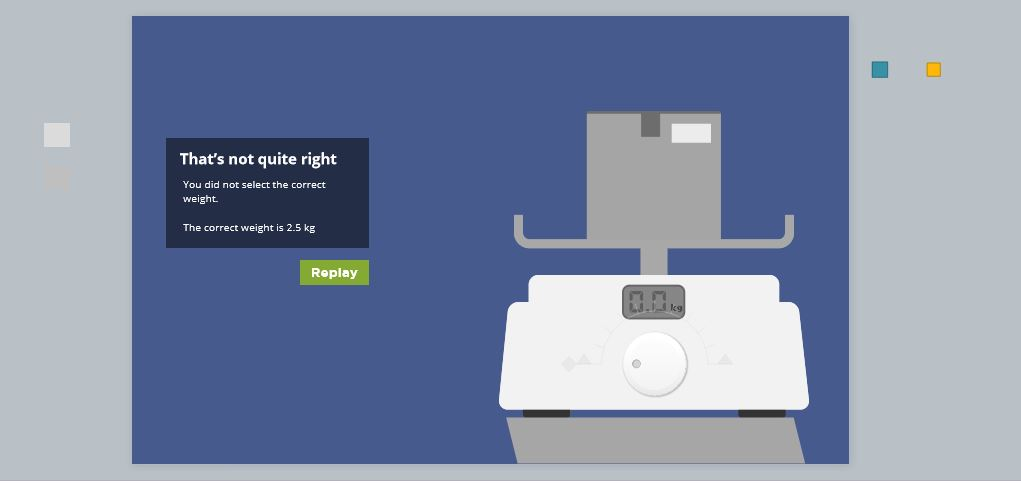In the previous post I shared steps on how to make a digital scale using the Dial facility in Articulate Storyline. In this post I am going to share on how to use the Digital Scale in a question slide/assessment slide. Here is the final output.

There is a small animation on the Incorrect response layer involving the scale.

Here are the steps:

Step 1Insert two shapes name them BT_correct and BT Incorrect respectively and place them outside the slide.

These boxes will be used when we insert the Freeform Question (Pick One).

Step 2

Insert Freeform QuestionSet the correct answer to BT_Correct and then incorrect answer to BT_Incorrect in the form view.Step 3

Set the Triggers

Now we need to ‘connect’ the Dial with the Freeform by using triggers. Since there isn’t any real question we just set the correct answer to 2.5kg.

Now we have to make a condition where BT correct is selected when the Dial equals to 2.5 kg or 5 in the Dial count.The other way round goes with the BT_incorrect shapeI am using a custom submit button (and hid the built in submit button by un-ticking it in the slide setting).By doing that the slide should be ready to use.

But for this demo we are adding small thing where the learner will be shown an animation to the correct answer if they answered wrong.

Step 4

We will need to offstage shapes, one is for when the learner answer less than the correct answer and the other one for when the learner answered more than the correct answer. Each shape has an animation path of 0.5s. For easy identifying I named them BT_add and BT_subtract. We then need to add conditions to mark when the buttons will move. For the add button it will move when the answer is (Variable Dial 2 in this case) is smaller.As for the BT subtract Motion path will move once the variable Dial 2 is bigger than than the correct answer (Variable Dial 2 > 5)If Dial 2 is less than 5, then we must add a value of 1 until it reaches five. that’s why we add another trigger:So once the animation is completed it will add 1 to Dial 2. We need to loop the animation so that it keeps adding until it reaches the correct answer and then stop. We stop the animation by adding a condition.The other way around with the BT Subtract button.  The button will move on its motion path when Dial 2 is larger than 5 and it will stop once Dial 2 = 5. The final triggers on the incorrect layer should be like this.here is the view on the incorrect layer with BT_Add and BT_subtract on the sideNow the Incorrect layer shall have a small animation showing the scale shifting to the correct answer. (Which would be a bit more fun than a static answer) – This treatment can also be used on slides with sliders.